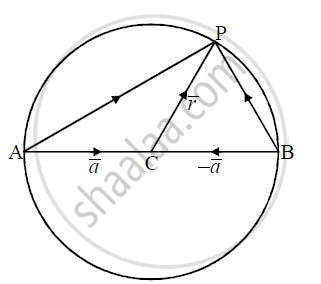# Using Vectors, Prove that Anglein a Semicircle is a Right Angle - Mathematics

Using Vectors, prove that angle in a semicircle is a right angle

#### Solution

Let sec AB be a diameter of a circle with centre C and P be any point on the circle other than A and B. Then

∠APB is an angle subtended on a semicircle

Let bar(AC) = bar(CB) = bar(a) and bar(CP) = bar(r)

Then |bar(a)| = |bar(r)| .... (1)

bar(AP) = bar(AC) +  bar(CP) = bar(a) + bar(r)= bar(r) + bar(a)

bar(BP) = bar(BC) + bar(CP) = -bar(CB) + bar(CP) = -bar(a) + bar(r)

:. bar(AP).bar(BP) = (bar(r) + bar(a)).(bar(r) - bar(a))

= bar(r).bar(r) - bar(r).bar(a) + bar(a).bar(r) - bar(a).bar(a)

= |bar(r)|^2 - |bar(a)|^2 = 0     ......(∵ bar(r).bar(a) = bar(a) .  bar(r))

:. bar(AP) ⊥ bar(BP) ∴ ∠APB is a right angle

Hence, the angle subtended on a semicircle is the right angle.Concept: Basic Concepts of Vector Algebra
Is there an error in this question or solution?